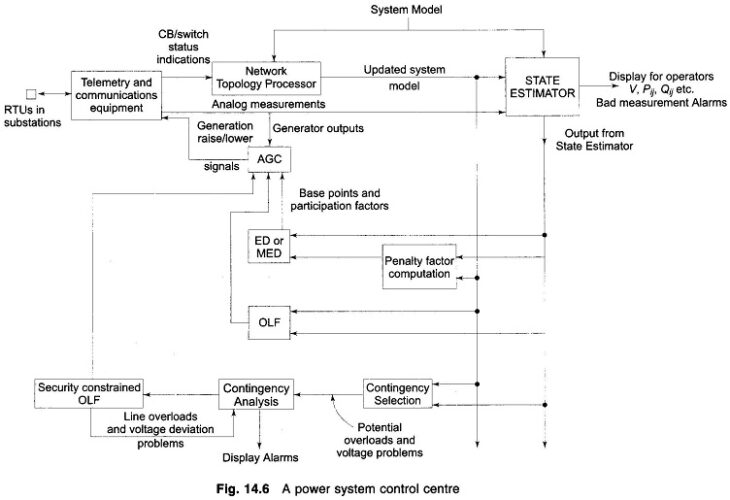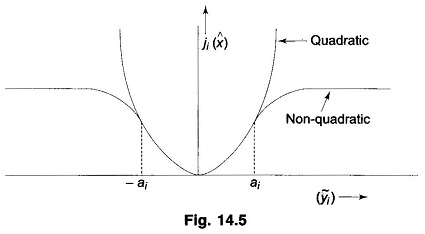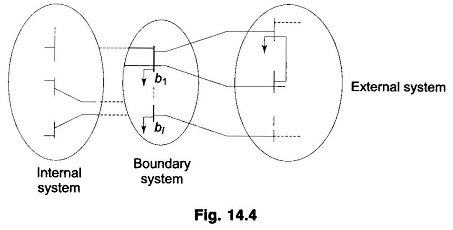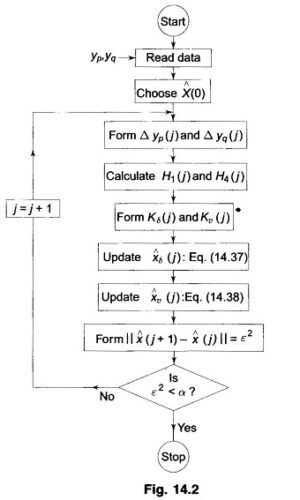# State Estimation of Power System

## Application of State Estimation in Power SystemApplication of State Estimation in Power System: Application of State Estimation in Power System – In real-time environment the state estimator consists of different modules such as network topology processor, observability analysis, state estimation and bad data processing. The network topology processor is required for all power system analysis. A conventional network topology program uses …

## Network Observability and Pseudo Measurements

Network Observability and Pseudo Measurements: A minimum amount of data is necessary for State Estimation (SE) to be effective. A more analytical way of determining whether a given data is enough for SE is called observability analysis. It forms an integral part of any real time state estimator.The ability to perform state estimation depends on …

## Bad Data Detection in State Estimation of Power SystemBad Data Detection in State Estimation of Power System: The ability to identify Bad Data Detection in State Estimation measurements is extremely valuable to a load dispatch centre. One or more of the data may be affected by malfunction­ing of either the measuring instruments or the data transmission system or,both. Transducers may have been wired …

## External System Equivalencing in Power SystemExternal System Equivalencing in Power System: One of the widely practiced methods used for computational simplification is to divide the given system into three subsystems as shown in Fig. 14.4. One of these is referred to as the ‘internal’ subsystem and consists of those buses in which we are really interested. The second subsystem consists …

## Tracking State Estimation of Power Systems

Tracking State Estimation of Power Systems: Tracking State Estimation of Power Systems is important for real time monitoring of the system. As is well known, the voltages of all real system vary randomly with time and should therefore be considered to be stochastic processes. It is thus necessary to make use of the sequential estimation …

## Static State Estimation in Power SystemsStatic State Estimation in Power Systems: Static State Estimation in Power Systems – As noted earlier, for a system with N buses, the state vector x may be defined as the 2N — 1 vector of the N — 1 voltage angles δ2, …, δN and the N voltage magnitudes V1, V2, …, Vn. The …

## Least Square Estimation Method

Least Square Estimation Method: As we are going to seen that, the problem of power system state estimation is a special case of the more general problem of estimation of a random vector x from the numerical values of another related random vector y with relatively little statistical information being available for both x and …

## Introduction to State Estimation in Power System

Introduction to State Estimation in Power System: Introduction to State Estimation in Power System plays a very important role in the monitoring and control of modem power systems. As in case of load flow analysis, the aim of State Estimation in Power System is to obtain the best possible values of the bus voltage magnitudes …

Scroll to Top